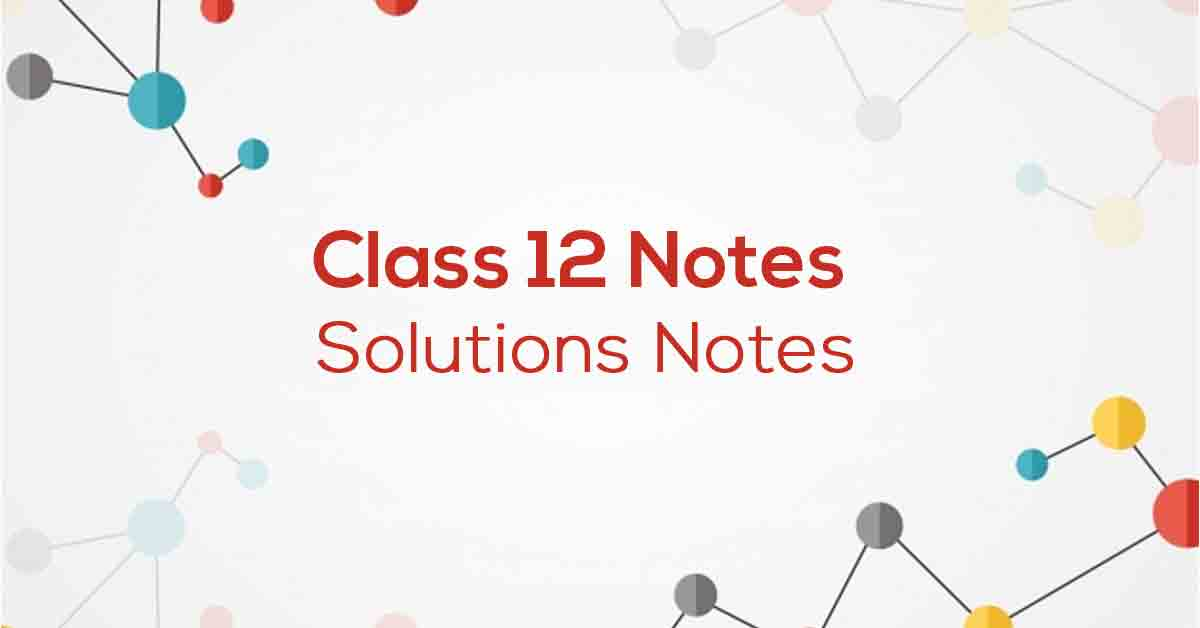Class 12th Chemistry Solutions NCERT Notes CBSE - 2023

# Solutions Class 12 Notes# Chapter 2 Solution Notes

The NCERT notes for Class 12 Chemistry Chapter 2 is solution-based. They are designed by experts according to the latest CBSE program to help students. Various important concepts are covered, such as solution types, solubility, binding properties, Raoult's law, and more. Vidyakul offers more than practice questions per sub-topic to help students prepare for the exam. Students coming to the exam must answer all these practical questions. Scroll down for more details. These NCERT notes will help students gain a competitive edge.

### Points to Remember

NCERT Notes for Grade 12 Chemistry Chapter 2: Important Points:-

• A solution is a homogeneous mixture of two or more non-chemically reactive substances whose composition can vary within certain limits.

• Henry's Law: The mass of a gas dissolved in a given volume of liquid at constant temperature is directly proportional to the pressure of the gas at equilibrium with the liquid or to a mixture of gases at In equilibrium with a liquid, the partial pressure of a Gas is proportional to the mole fraction of a gas in a solution.

• Raoult's law in its general form can be stated as, for any solution, the partial vapor pressure of each volatile component in the solution is proportional to its molar fraction.

• Solvents and solutes. In a binary solution, the component in smaller amounts is known as the Soluble, while the component in larger quantities is known as the Solvent. If water is the solvent, the solution is called an aqueous solution.

### Topics and Sub-Topics

Click on the topic-wise links provided in the table below to get access to the NCERT Chemistry Chapter 2 chapter-wise important questions:

Section Name

Topic Name

Description

2.1

Types of Solutions

A solution comprises a solute and a solvent. It is defined as a homogeneous mixture of two or more than two substances. They can be classified into three types:

• Solid solutions

• Gaseous solutions

• Liquid solutions

2.2

Methods of Expressing Concentration of Solutions

2.3

Solubility

2.4

Vapour Pressure of Liquid Solutions

2.5

Raoult’s Law

The lowering of the vapour pressure of the solvent is ruled by Raoult’s law. The law states that the relative lowering of the vapour pressure of the solvent over a solution and the mole fraction of a non-volatile solute present in the solution is equal. It is expressed as:

Ptotal= p10x1+p20x2

2.6

Ideal and Non-ideal Solutions

## Ideal Solutions

When a solution obeys Raoult’s law over the entire range of concentrations it is called an ideal solution. Types of deviations from Raoult’s law:

• Positive deviations

• Negative deviations

2.7

Colligative Properties

2.8

Elevation in Boiling Point of The Solvent

2.9

Depression in Freezing Point of The Solvent

2.1

Osmosis and Osmotic Pressure of The Solution

2.11

Van’t Hoff’s Factor

1.

Download Vidyakul App for more Important videos, PDF's and Free video lectures.

### Few Important Questions

• What are the different types of solutions?

The 3 main types of solutions are: 1. Solid 2. Liquid 3. Gas

• What are ‘Ideal solutions’?

When a solution obeys Raoult’s law over the entire range of concentrations it is called an ideal solution.

• What is ‘Molarity’?

Molarity or molar concentration, is the concentration of a solution measured as the number of moles of solute per liter of solution.

### Practice Questions

1. What is mole fraction?

2. Explain the role of molecular interaction in a solution of water and alcohol?

3. Give the statement of Henry’s law. List some applications.

4. What is a solution? Explain the different types of solutions.

5. What is molarity?